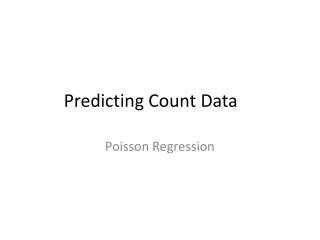DownloadDownload PresentationPredicting Count Data

# Predicting Count Data

Download Presentation## Predicting Count Data

- - - - - - - - - - - - - - - - - - - - - - - - - - - E N D - - - - - - - - - - - - - - - - - - - - - - - - - - -
##### Presentation Transcript

1. Predicting Count Data Poisson Regression

2. Review: Confusing Statistical Terms General Linear Model (GLM) -Anything that can be written like this: -Solved using ordinary least squares -Assumptions revolve around the Normal Dist. Generalized Linear Model -Anything that can be written like this: -Solved using maximum likelihood -Assumptions use many different distributions

3. Remember: Why These Models? • Linear Regression: Assuming normal errors around the predicted score • When we violate this assumptions, our estimates of the distributions of the B’s are incorrect • Also…in some case our estimates of the effect size are inaccurate (usually too small)

4. Linear Regression • Linear regression is really a predictive model before anything else. (The statistical aspect is extra). B1 B0

5. Count Data

6. Examples • (Criminal Justice) Number of offenses per year • (Domestic Violence) Number of DV events per person • (Epidemiology) Number of seizures per week

7. Count Data • This type of data can only have discrete values that are greater than or equal to zero. • In situations, this data follows the Poisson Distribution

8. Poisson Distribution • The Poisson random variable is defined by one parameter: the mean (μ) • It has the strong assumption that the mean is equal to the variance μ=σ

9. Poisson Regression • In this model, instead of predicting mean of a normal distribution, you are predicting the mean of a Poisson distribution (given some predictors)

10. Fundamental Equation • In linear regression: • In Poisson regression:

11. Assumptions • In your outcome variable (Y), the mean equals the variance. (There is a test for this) • For violations you can use Negative Binomial…which is just a Poisson where the variance is separate from the mean. • Observations are independent (as with most analyses) • And, basically, that the predictive model makes sense ( )

12. Interpreting Parameters • Like logistic, we have to interpret the EXP(B) • (This is the notation for ) • Instead of an odds ratio, this is a relative risk ratio: it is the additional rate given a one unit increase in X • 1 is the null hypothesis • 1.2 would be an increase of .2 in the relative rate for a one unit increase

13. Really, why the trouble? • Turns out that not using Poisson isn’t the worst thing ever. • Actually get alpha deflation • BUT- Many journals that are used to this kind of data will reject articles that do not use the proper technique